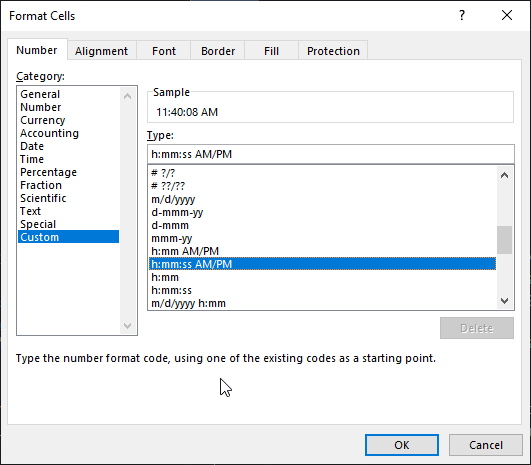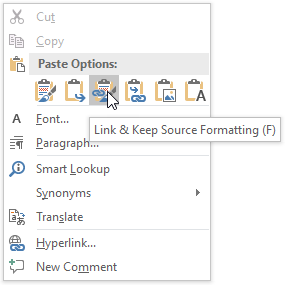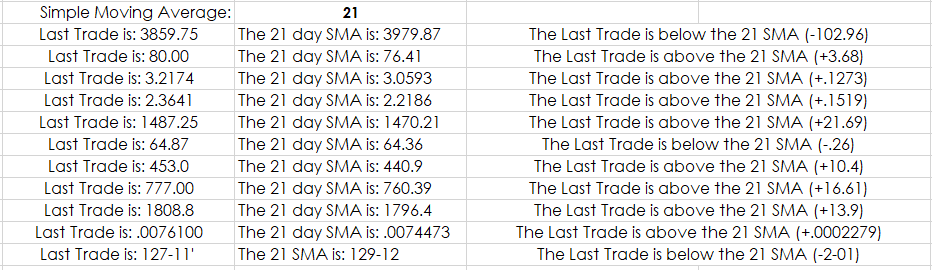# Excel's Text Function

Microsoft® Excel has a Text function which is useful for combining text with market data. For example, you may want to have your Excel dashboard display in the same cell the percent net change value of a market with the word “Higher” or “Lower”. You cannot in a cell use the RTD formula for percent net change combined with the text.

The solution is to use Excel’s Text function and concatenate. The Text concatenation operator in Excel is the ampersand symbol (&). You can use it to join two or more text strings in a single string. First, a look at the Text function, which is “=TEXT(Value, Format).”

The Text function converts a number to text with your choice of formatting. This table of examples is from Microsoft Excel’s Help.

Formula Description
=TEXT(1234.567,"\$#,##0.00") Currency with a thousands separator and 2 decimals, such as \$1,234.57. Note that Excel rounds the value to 2 decimal places.
=TEXT(TODAY(),"MM/DD/YY") Today’s date in MM/DD/YY format, such as 03/14/22
=TEXT(TODAY(),"DDDD") Today’s day of the week, such as Monday
=TEXT(NOW(),"H:MM AM/PM") Current time, like 1:29 PM
=TEXT(0.285,"0.0%") Percentage, such as 28.5%
=TEXT(4.34 ,"# ?/?") Fraction, such as 4 1/3
=TRIM(TEXT(0.34,"# ?/?")) Fraction, such as 1/3. Note this uses the TRIM function to remove the leading space with a decimal value.
=TEXT(12200000,"0.00E+00") Scientific notation, like 1.22E+07
=TEXT(1234567898,"[<=9999999]###-####;(###) ###-####") Special (Phone number), such as (123) 456-7898

So, in our case we wanted to display in the same cell the text version of a market’s percent net change with the word Higher:

`=TEXT(RTD("cqg.rtd",,"ContractData","GCE","PerCentNetLastTrade",,"T")/100,"0.00%")&" Higher”`

The Value used in the Text function is the RTD formula for percent net change divided by 100 and the format is percentage using two decimal places (“0.00%”) and the & connects the word “ Higher.” Notice the space following the open quote so there is a space between the percent net change and Higher.

We can use an If Then Excel function to check if the percent net change is positive or negative to display “ Higher” or “ Lower”:

`=IF(RTD("cqg.rtd",,"ContractData","GCE","PerCentNetLastTrade",,"T")/100>0,TEXT(RTD("cqg.rtd",,"ContractData","GCE","PerCentNetLastTrade",,"T")/100,"0.00%")&"Higher",TEXT(RTD("cqg.rtd",,"ContractData","GCE","PerCentNetLastTrade",,"T")/100,"0.00%")&" Lower")`

One trick to finding the proper formatting code is to right-click a cell, choose Format, choose Number and then Custom. For example, if you want the current time to be displayed you can use the RTD function “Line Time.” To display this: “The time is: 12:55:51 PM” you use this:

`="The time is: "&TEXT(MOD(RTD("cqg.rtd",,"SystemInfo","Linetime"),1),"h:mm:ss AM/PM")`

The format code was copied from the Type box and pasted into the format parameter surrounded by quotes.

The Excel Mod function is used in the formula applied to Line Time because Line Time includes the date to the left of the decimal and the time is to the right of the decimal. Divide by “1” returns just the time value.If you had a regular report you produced in Word you can link the Excel Spreadsheet to the Word document by selecting the cell in Excel, choose copy, and in Word choose to Paste Link. The text values will automatically update in Word.The downloadable Excel sample contains numerous examples of combining text with market and study values brought into Excel using RTD. This sample is using the sample from the post titled: “Excel Functions for Automatically Formatting Market PricesThat post details the steps to automatically format prices based on the tick sized and those same steps are utilized in this sample.

The third column in the image above displays the difference between the last price and the moving average. The series of If Then functions is complicated because if the contract is a fixed income instrument then Dollar Fraction function is used to convert the decimal price to 32nds.

`=DOLLARFR(101.25,32) =101.08`

But, the decimal is replaced with a dash in the formulas.

101.08 = 101-08